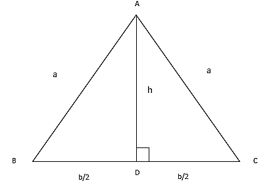# Java Program To Find Area Of Isosceles Triangle

Java program to find the area of an Isosceles triangle or to calculate the area of an isosceles triangle. Before we discuss the programming code what is an isosceles triangle? And what are the main functions of an isosceles?

What is an isosceles triangle?

An isosceles triangle is a triangle where any two sides of a triangle should be equal both in terms of length and angles. Below is the sample figure.

What is the formula to find out the area of an isosceles triangle?

Since the area of a normal triangle and isosceles have the same formula. And, the same program is applicable when it comes to the coding part in java. Here goes the formula, make sure to note down the base and height values. The base is opposite to the two-sided lengths or angles which are in equal.[ B/4√((4*a*a)-(b*b)) ]

You can write the code in different formats, one of the format here as follows, consider it has # sample method -1

Output:

As normal, we can calculate the area of an isosceles triangle with the standard formula, in order to make the unique one. we write the java program in a different method. check it out the above code so that you will get an idea.

There may be so many different ways in order to calculate the are the area of an isosceles triangle, here the math formula that we are used as ” [ B/4√((4*a*a)-(b*b)) ]  “.

Here goes the complete explanation of the above code :

1) No need to say about the ” import java.util.Scanner; ” you may have an idea about that.

2)

– Allocating a particular name for the following code.

3)

– The code starts executing from here.

4)

– The scanner is a class, which is inbuilt in JDK package which can read the inputs from the system, whereas system.in is to read the inputs from the same system that you were using.

5)

– instruction for the user to  Enter the value of side of triangle .the value is same for two sides.

6)

it takes first input and stores value in variable a.

7)

Same as follows for the: side 3

8)

– java formula to find the area of an isosceles

9)

-Output displayed on the screen, once you started compiling and executing the values.

So, that’s the complete explanation of how the java program code works in order to find the area of an isosceles triangle. If you have any doubt related to this section do comment here, we are glad to help you out.

Moreover, here is the #sample method-2, you can even compile and execute the program here!

In the above code, we are not using the scanner function, where scanner function is an inbuilt package that read the inputs from the system, in this case, scanner function is not available on online compiler, for that purpose we are using the command line arguments methodology to get input from the user to do this  correctly we need some function parseXXX() method   to will convert string to number format.

Note: While you were using the arguments to read the input values, enter the values from ” 1-10 space 1-10 “. The first value is the length of the two same sides, and the next one is a side of another length.

You can also use the “Int” instead of ” Double”, where double can represent the values even after decimal points to, whereas in Int will won’t display the values.

If you have any doubts related to this section do comment here, we are glad to help you out related to this query.

x

## X Star Pattern Java Program – Patterns

Java program to print X star pattern program – We have written the below print/draw ...